# Dead Variable Analysis

In this post, I implement dead variable analaysis, a static analysis that finds dead variables. Dead variable analysis is useful because it allows a compiler to reclaim dead resources. I'm implementing this analysis and writing about it to help me study data flow analysis, a general form of static analysis that includes dead variable analysis. I'm reading about it in Khedker, Sanyal, and Karkare's Data Flow Analysis Theory and Practice.

## What is control flow analysis?

Control flow analysis uses control graphs to statically analyze a program. Control flow graphs represent the possible paths of program flow. A conditional with a true branch and a false branch, for example, flows into two different paths. This can bee seen in 1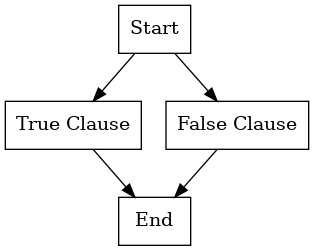Figure 1: This control flow graph corresponds to a conditional. There are two clauses: a true and false clause.

A loop flows either forwards, the loop ending, or back to an early part of the program, the loop starting a new iteration. This can be seen in 2.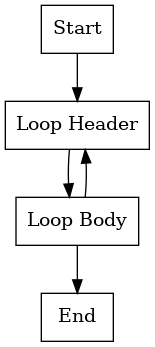Figure 2: This control flow graph corresponds to a loop. There is an initial body for updating iteration variables and checking whether to continue the loop.

The simpelest flow is one statement reaching another.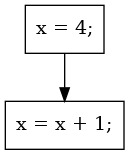Figure 3: This control flow graph corresponds to two consecutive statements. Each statement has its own control flow graph node.

Consecutive statements can be combined into groups called basic blocks. The statements from figure 3 are combined into a basic block in fig:basiscblock.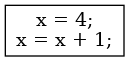Figure 4: This control flow graph corresponds to two consecutive statements, the same statements from 3, but in this control flow graph both statements belong to the same node.

Grouping individual statements into basic blocks is preferrable because grouping reduces the size of the control flow graph. Grouping individual statements into basic blocks is possible because the path through a series of consecutive blocks always has the same effect because there's only ever one path.

Control flow analyis is used to determine static properties of a program. An example of this is searching for dead variables. If a variable is live, then memory must be set aside for it. However, at the end of the program the memory can be reclaimed. This is because the variable will no longer be used; the variable is dead, because the program is over. If the last statement of the program before the basic block doesn't use that variable, then memory can be reclaimed before that last statement. If the third to last statement and every possible path from it to the end of the program does not use the variable, then the memory can be reclaimed before the third statement.

datacanalwaysbereclaimed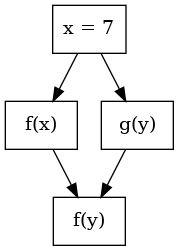Figure 5: In this control flow graph, the variable `x` is dead after the second node. After the second node, the memory allocated for `x` can be reclaimed and overwritten.

Note that for a variable to be dead at a node, every path from that node to the end cannot use the variable. Branches and jumps create multiple paths.Figure 6: In this control flow graph, the variable `x` is dead on one path, but not the other. We can't be sure the variable is dead before the program runs, because we don't know which path the program will take.

There are many types of data flow analysis. An algorithm to detect dead variable analysis will be shown.

## Program Structure

First we need a program to test on. Our example program will be our test input and be what the rest of our code is built around parsing.

We'll start with a graph data structure. This will represent our control flow graph. The nodes will be basic blocks. We'll use object references to the basic blocks as node identities.

```(defclass directed-graph ()
((edges :initform (make-hash-table))))

(defmethod add-node ((graph directed-graph) new-node)
(with-slots (edges) graph
(setf (gethash new-node edges)
(gethash new-node edges nil))))

(defmethod add-edge ((graph directed-graph) source-node destination-node)
;; Ensure nodes exist.
;; Create edge. (Doesn't check if edge already exist.)
(with-slots (edges) graph
(push destination-node (gethash source-node edges))))
```

The following functions will allow us to query our graph:

• `nodes` will get a list of the nodes
• `successors` will take a node and give all the nodes it points to
• `predecessors` will take a node and give all the nodes pointing to it.
```(defmethod nodes ((graph directed-graph))
(with-slots (edges) graph
(loop for node being the hash-keys of edges
collect node)))

(defmethod succesors ((graph directed-graph) node)
(with-slots (edges) graph
(gethash node edges nil)))

(defmethod predecessors ((graph directed-graph) node)
(with-slots (edges) graph
(loop for key being the hash-keys of edges
if (member node (gethash key edges))
collect key)))
```

Now we need our program. We'll be using pseudocode from Kedker Sanyal and Karkare's book.

examplepseudoCode

First we'll need a basic block. These will represent our graph nodes. If a node only has one statement, we'll still wrap it in a one statement basic block.

```(defclass basic-block ()
((statements :initform (list) :initarg :statements)
(name :initform nil :initarg :name :reader basic-block-name)))

(defmethod print-object ((item basic-block) out)
(print-unreadable-object (item out :type t)
(with-slots (name) item
(format out "~s" name))))
```

Next we need statements. There are two kinds of statements:

• Assignment statements.
• Expression statements.

Assignment statements modify variables. Expression statements perform a calculation and throw it away.

```(defclass statement () ())

(defclass assignment-statement (statement)
((left-value :initarg :left-value)
(right-value :initarg :right-value)))

(defclass expression-statement (statement)
((expression :initarg :expression)))
```

Finally, we need expressions. There are three kinds:

• Binary operations.
• Literals.
• Function calls.
• Variable references.
```(defclass expression () ())

(defclass binary-operation-expression (expression)
((operator :initarg :operator)
(operand-1 :initarg :operand-1)
(operand-2 :initarg :operand-2)))

(defclass literal-expression (expression)
((value :initarg :value)))

(defclass function-call-expression (expression)
((function :initarg :function)
(arguments :initarg :arguments)))

(defclass variable-expression (expression)
((identifier :initarg :identifier)))
```

With this, we have enough to describe a simple language and the control flow graph of programs. Next, we'll create a small program.

## A Sample Program

We'll be copying Kedker, Sanyal, and Karkare's example program.

To make the program easier to write, lets define some helpers.

```(defun make-basic-block (name &rest statements)
(make-instance 'basic-block :statements statements :name name))

(defun make-assignment (left-value right-value)
(make-instance 'assignment-statement
:left-value left-value
:right-value right-value))

(defun make-variable (identifier)
(make-instance 'variable-expression :identifier identifier))

(defun make-literal (value)
(make-instance 'literal-expression :value value))

(defun make-binary-operation (operator operand-1 operand-2)
(make-instance 'binary-operation-expression
:operator operator
:operand-1 operand-1
:operand-2 operand-2))

(defun make-function-call (function &rest arguments)
(make-instance 'function-call-expression
:function function
:arguments arguments))

(defun make-expression (expression)
(make-instance 'expression-statement
:expression expression))
```

Now lets define the basic blocks.

```(defparameter *n-1*
(make-basic-block
'n-1
(make-assignment
(make-variable 'b)
(make-literal 4))
(make-assignment
(make-variable 'a)
(make-binary-operation '+ (make-variable 'b) (make-variable 'c)))
(make-assignment
(make-variable 'd)
(make-binary-operation '* (make-variable 'a) (make-variable 'b)))))

(defparameter *n-2*
(make-basic-block
'n-2
(make-assignment (make-variable 'b)
(make-binary-operation '- (make-variable 'a) (make-variable 'c)))))

(defparameter *n-3*
(make-basic-block
'n-3
(make-assignment (make-variable 'c)
(make-binary-operation '+ (make-variable 'b) (make-variable 'c)))))

(defparameter *n-4*
(make-basic-block
'n-4
(make-assignment (make-variable 'c)
(make-binary-operation '* (make-variable 'a) (make-variable 'b)))
(make-expression
(make-function-call 'f (make-binary-operation '- (make-variable 'a) (make-variable 'b))))))

(defparameter *n-5*
(make-basic-block
'n-5
(make-assignment (make-variable 'd)
(make-binary-operation '+ (make-variable 'a) (make-variable 'b)))))

(defparameter *n-6*
(make-basic-block
'n-6
(make-expression
(make-function-call 'f
(make-binary-operation '+ (make-variable 'b) (make-variable 'c))))))

(defparameter *n-7*
(make-basic-block
'n-7
(make-expression
(make-function-call 'g
(make-binary-operation '+ (make-variable 'a) (make-variable 'b))))))

(defparameter *n-8*
(make-basic-block
'n-8
(make-expression
(make-function-call 'h (make-binary-operation '- (make-variable 'a) (make-variable 'c))))
(make-expression
(make-function-call 'f (make-binary-operation '+ (make-variable 'b) (make-variable 'c))))))
```

And finally, stitch them together in a graph.

```(let ((graph (make-instance 'directed-graph)))
(add-edge graph *n-1* *n-2*)
(add-edge graph *n-2* *n-8*)
(add-edge graph *n-1* *n-3*)
(add-edge graph *n-3* *n-4*)
(add-edge graph *n-4* *n-7*)
(add-edge graph *n-7* *n-8*)
(add-edge graph *n-3* *n-5*)
(add-edge graph *n-5* *n-6*)
(add-edge graph *n-6* *n-7*)
(add-edge graph *n-7* *n-3*)
(add-edge graph *n-6* *n-5*)
(defparameter *graph* graph))
```

This is a complicated enough program to showcase our dead variable analysis

## Analysis

Control flow analysis is based on generating four different sets:

• Gen
• Kill
• In
• Out

Generate and Kill are sets that describe individual basic blocks. Generate and kill are calculated once and then reused through the analysis. Their values never change.

In and Out are also sets that describe individual basic blocks, but they are calculated several times. When the analyzer reaches a fix point, in and out wont change, then the analysis is done.

To help us calculate the generate and kill sets, we'll define the `get-defined` and `get-used` helper functions.

`get-defined` will collect all the variables defined in a basic block. It just walks the statements and collects the variable in the left side of each statement.

```(defmethod get-defined ((graph directed-graph))
(loop for node in (nodes graph)
append (get-defined node)))

(defmethod get-defined ((statement statement))
nil)

(defmethod get-defined ((basic-block basic-block))
(with-slots (statements) basic-block
(loop for statement in statements
append (get-defined statement))))

(defmethod get-defined ((statement assignment-statement))
(if (typep statement 'assignment-statement)
(with-slots (left-value) statement
(with-slots (identifier) left-value
(list identifier)))
nil))
```

Get used collects variable occurences that aren't lvalues.

```(defmethod get-used ((statement assignment-statement))
(with-slots (right-value) statement
(get-used right-value)))

(defmethod get-used ((statement expression-statement))
(with-slots (expression) statement
(get-used expression)))

(defmethod get-used ((expression function-call-expression))
(with-slots (arguments) expression
(loop for argument in arguments
append (get-used argument))))

(defmethod get-used ((expression literal-expression))
nil)

(defmethod get-used ((expression binary-operation-expression))
(with-slots (operand-1 operand-2) expression
(append (get-used operand-1)
(get-used operand-2))))

(defmethod get-used ((expression variable-expression))
(with-slots (identifier) expression
(list identifier)))
```

With these two methods we'll define generate and kill

Generate will

```;; Generate

(defmethod generate ((analysis-type (eql :dead-variable)) (statement assignment-statement))
(with-slots (left-value) statement
(with-slots (identifier) left-value
(list identifier))))

(defmethod generate ((analysis-type (eql :dead-variable)) (statement expression-statement))
(get-used statement))

(defmethod generate ((analysis-type (eql :dead-variable)) (expression function-call-expression))
(get-used expression))

(defmethod generate ((analysis-type (eql :dead-variable)) (expression literal-expression))
(get-used expression))

(defmethod generate ((analysis-type (eql :dead-variable)) (expression binary-operation-expression))
(get-used expression))

(defmethod generate ((analysis-type (eql :dead-variable)) (expression variable-expression))
(get-used expression))
```

There is one last method, the trickiest one. In simple control flow analysis graphs, there are no basic blocks. Put another way, each basic blocks only contains one statement. This simplifies analysis, but requires larger graphs, so consecutive statements are often coalesced into one block. Unfortunately, calculating `gen` and `kill` for a single block becomes much more complicated. A simplified version of the control flow analysis algorithm must be run on the simple block.

Here, we say that a basic block generates the every variable definition that does not precede a variable use within the block. We calculate this by walking backwards through the statements.

```(defmethod generate ((analysis-type (eql :dead-variable)) (basic-block basic-block))
(with-slots (statements) basic-block
(reduce (lambda (generate-accumulator new-statement)
(remove-duplicates
(append (set-difference (get-defined new-statement)
(get-used new-statement))
(set-difference generate-accumulator
(get-used new-statement)))))
(reverse (copy-seq statements))
:initial-value nil)))
```

In the following, the use of `a` precedes a modification of `a` so `a` is not in the gen set.

exampleause.

There is a special case. In assignment, the `rvalue` is calculated before the `lvalue` is modified; the use of the `lvalue` precedes the modification of the `rvalue`. If the `lvalue` variable of an assignment appears in the `rvalue` of the same assignment, the variable is not in the gen set.

examplea=a+1.

Now that generate is defined, we can generate kill. The kill just looks for any variable occurences besides a `lvalue`.

```(defmethod kill ((analysis-type (eql :dead-variable)) (statement assignment-statement))
(with-slots (right-value) statement

(defmethod kill ((analysis-type (eql :dead-variable)) (statement expression-statement))
(with-slots (expression) statement

(defmethod kill ((analysis-type (eql :dead-variable)) (expression function-call-expression))
(with-slots (arguments) expression
(loop for argument in arguments
append (kill :dead-variable argument))))

(defmethod kill ((analysis-type (eql :dead-variable)) (expression literal-expression))
nil)

(defmethod kill ((analysis-type (eql :dead-variable)) (expression binary-operation-expression))
(with-slots (operand-1 operand-2) expression
(append (kill :dead-variable operand-1)

(defmethod kill ((analysis-type (eql :dead-variable)) (expression variable-expression))
(with-slots (identifier) expression
(list identifier)))
```

With this, we have the gen and kill sets. Now we need to calculate the in and out sets. The in and out set of each basic-block is defined by these simultanious equations. Notice that they're mutually recursive. To find the in and out sets that make these equations true, we will iteratively calculate them until we hit a fixed point.

```(defun dead-variable-analysis (graph)
(let ((generate-table (generate-table :dead-variable graph))
(kill-table (kill-table :dead-variable graph))
(in-table (make-hash-table))
(out-table (make-hash-table)))
(let ((every-variable (remove-duplicates (get-defined graph))))
(loop for node in (nodes graph)
do (setf (gethash node in-table) every-variable
(gethash node out-table) every-variable)))
(loop for i upto 10 ; This should stop when there are no more in/out changes.
do (loop for node in (nodes graph)
do (progn
(when (succesors graph node)
(setf (gethash node out-table)
(remove-duplicates
(reduce #'intersection
(loop for succesor-node in (succesors graph node)
collect (gethash succesor-node in-table))))))
(setf (gethash node in-table)
(remove-duplicates
(append (set-difference (gethash node out-table)
(gethash node kill-table))
(gethash node generate-table)))))))
(values in-table out-table)))
```

We'll add a utility function to print out the in and out sets.

```(defun print-hash-table (hash-table)
(let ((sorted-keys (sort (loop for key being the hash-keys of hash-table collect key)
#'string>
:key #'basic-block-name)))
(loop for key in sorted-keys
do (format t "~a:~a~%" key (sort (gethash key hash-table) #'string<)))))
```

Our results.

```CONTROL-FLOW> (multiple-value-bind (in out) (dead-variable-analysis *graph*)
(print-hash-table in)
(format t "~%")
(print-hash-table out))
#<BASIC-BLOCK N-8>:(D)
#<BASIC-BLOCK N-7>:(D)
#<BASIC-BLOCK N-6>:(D)
#<BASIC-BLOCK N-5>:(D)
#<BASIC-BLOCK N-4>:(C D)
#<BASIC-BLOCK N-3>:(D)
#<BASIC-BLOCK N-2>:(B D)
#<BASIC-BLOCK N-1>:(A B D)

#<BASIC-BLOCK N-8>:(A B C D)
#<BASIC-BLOCK N-7>:(D)
#<BASIC-BLOCK N-6>:(D)
#<BASIC-BLOCK N-5>:(D)
#<BASIC-BLOCK N-4>:(D)
#<BASIC-BLOCK N-3>:(D)
#<BASIC-BLOCK N-2>:(D)
#<BASIC-BLOCK N-1>:(D)
NIL
```

Lets check if this makese sense. The variable `d` is dead before and after every node, but we see that it's never used; `d` is assigned to but the value is never used. The out set of `*n-8*` includes every variable. This makes sense because it's the end of the program; none of the variables will be used again. The in set to `*n-8*` also makes sense. `d` is dead, but `a`, `b`, and `c` are all used within `*n-8*`. In `*n-4*`, `c` is dead because `*n-3*` modifies it.

## Conclusion

In this blog post